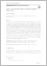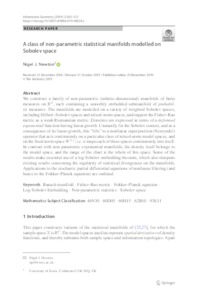# A Class of Non-Parametric Statistical Manifolds modelled on Sobolev Space

Newton, Nigel J (2019) 'A Class of Non-Parametric Statistical Manifolds modelled on Sobolev Space.' Information Geometry, 2. 283 - 312. ISSN 2511-2481Preview
Text
Newton2019_Article_AClassOfNon-parametricStatisti.pdf - Published Version
Available under License Creative Commons Attribution.

Download (469kB) | Preview

## Abstract

We construct a family of non-parametric (infinite-dimensional) manifolds of finite measures on Rd. The manifolds are modelled on a variety of weighted Sobolev spaces, including Hilbert-Sobolev spaces and mixed-norm spaces. Each supports the Fisher-Rao metric as a weak Riemannian metric. Densities are expressed in terms of a deformed exponential function having linear growth. Unusually for the Sobolev context, and as a consequence of its linear growth, this "lifts" to a nonlinear superposition (Nemytskii) operator that acts continuously on a particular class of mixed-norm model spaces, and on the fixed norm space W²'¹ i.e. it maps each of these spaces continuously into itself. It also maps continuously between other fixed-norm spaces with a loss of Lebesgue exponent that increases with the number of derivatives. Some of the results make essential use of a log-Sobolev embedding theorem. Each manifold contains a smoothly embedded submanifold of probability measures. Applications to the stochastic partial differential equations of nonlinear filtering (and hence to the Fokker-Planck equation) are outlined.

Item Type: Article math.PR, math.PR, 60D05, 62B10 (Primary) 46N30, 60H15, 93E11 (Secondary) Faculty of Science and Health > Computer Science and Electronic Engineering, School of Elements 03 Oct 2019 11:07 15 Jan 2020 16:15 http://repository.essex.ac.uk/id/eprint/25448

### Actions (login required)View Item